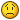## Recommended Posts

hi,

I found out that the anchor.y is inaccurate for BitmapText. When I set it to 0.5, it doesn't align to center,

but if I set anchor.y to 0 and add half of it's height to y, it will correctly locate at center.

##### Share on other sites

I dont know about thatMy telepathic abilities dont give anything on it, so please post it in in "issues" and with a fiddle. Unless @themoonrat knows?

##### Share on other sites

What tool did you use for your font?

Open your .fnt or .xml file.

Make sure that lineHeight is equal to size.

Example:

``````<info face="Font" size="64" bold="0" italic="0" charset="" unicode="" stretchH="100" smooth="1" aa="1" padding="2,2,2,2" spacing="0,0" outline="0"/>
<common lineHeight="64" base="55" scaleW="2048" scaleH="512" pages="1" packed="0"/>``````

##### Share on other sites

lineHeight it is, my lineHeight is not the same as char height before(as shown). So If I want the anchor.y based on char height, I need to make sure lineHeight is set to the same value,
and as @ivan.popelyshev said, be aware of offset.

``````<font>
<info face="num_02" size="32" bold="0" italic="0" charset="" unicode="1" stretchH="100" smooth="1" aa="1" padding="0,0,0,0" spacing="1,1" outline="0"/>
<common lineHeight="32" base="26" scaleW="256" scaleH="256" pages="1" packed="0" alphaChnl="0" redChnl="4" greenChnl="4" blueChnl="4"/>
<pages>
<page id="0" file="num_02.png" />
</pages>
<chars count="11">
<char id="48" x="89" y="0" width="40" height="54" xoffset="0" yoffset="0" xadvance="40" page="0" chnl="15" />
<char id="49" x="130" y="0" width="40" height="54" xoffset="0" yoffset="0" xadvance="40" page="0" chnl="15" />
<char id="50" x="171" y="0" width="40" height="54" xoffset="0" yoffset="0" xadvance="40" page="0" chnl="15" />
<char id="51" x="212" y="0" width="40" height="54" xoffset="0" yoffset="0" xadvance="40" page="0" chnl="15" />
<char id="52" x="0" y="55" width="40" height="54" xoffset="0" yoffset="0" xadvance="40" page="0" chnl="15" />
<char id="53" x="41" y="55" width="40" height="54" xoffset="0" yoffset="0" xadvance="40" page="0" chnl="15" />
<char id="54" x="82" y="55" width="40" height="54" xoffset="0" yoffset="0" xadvance="40" page="0" chnl="15" />
<char id="55" x="123" y="55" width="40" height="54" xoffset="0" yoffset="0" xadvance="40" page="0" chnl="15" />
<char id="56" x="164" y="55" width="40" height="54" xoffset="0" yoffset="0" xadvance="40" page="0" chnl="15" />
<char id="57" x="205" y="55" width="40" height="54" xoffset="0" yoffset="0" xadvance="40" page="0" chnl="15" />
</chars>
</font>``````

##### Share on other sites

So, is that solved? if not, its easier for you to find out what's wrong in https://github.com/pixijs/pixi.js/blob/dev/src/extras/BitmapText.js , because I'm not familiar with that code.

##### Share on other sites

It's resolved. anchor.y based on lineHeight, and in my .fnt file, I didn't set it correctly.
Thanks @ivan.popelyshev

## Create an account or sign in to comment

You need to be a member in order to leave a comment

## Create an account

Sign up for a new account in our community. It's easy!

Register a new account